Publicité

# Python基本資料運算

14 Aug 2021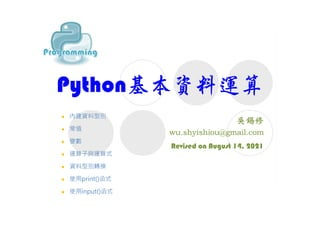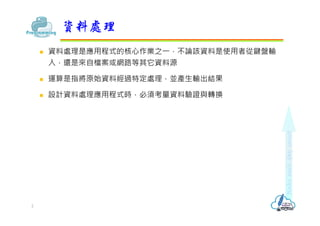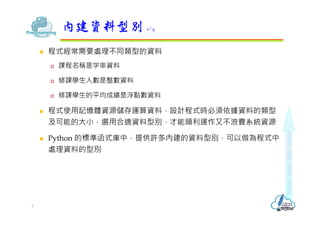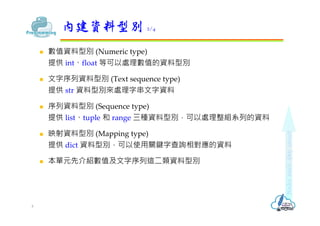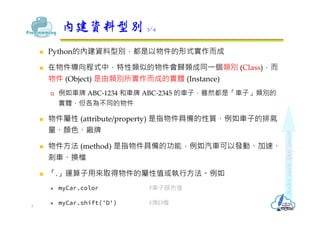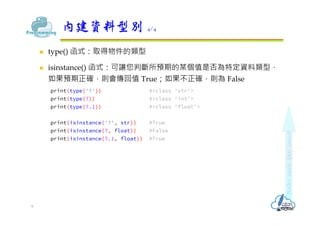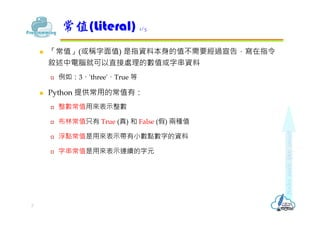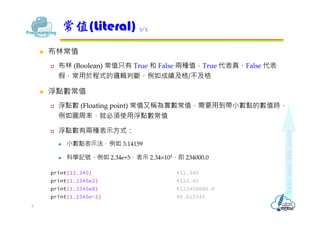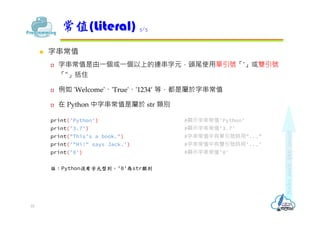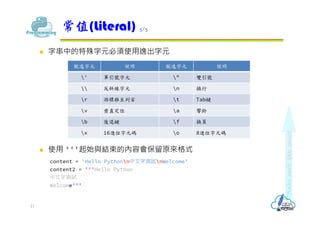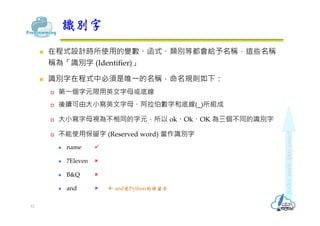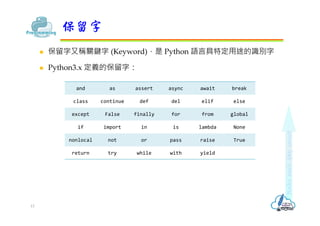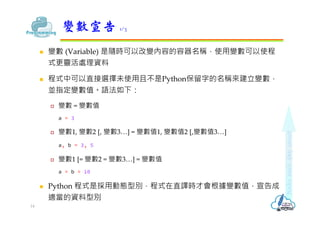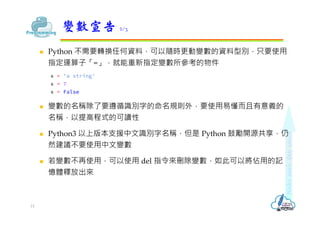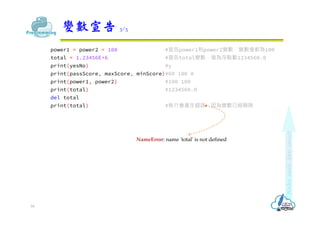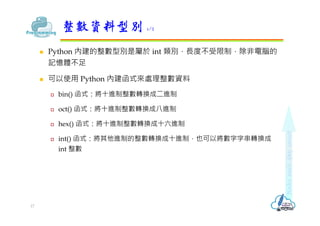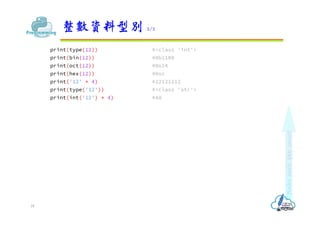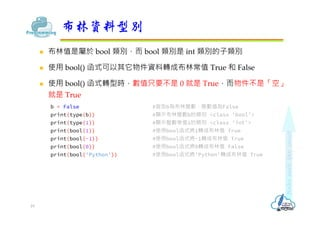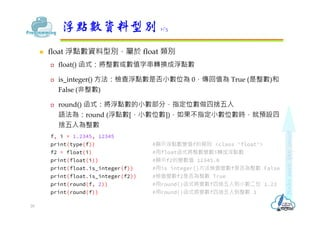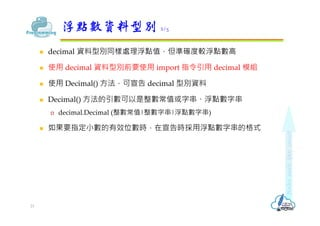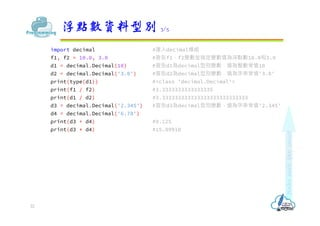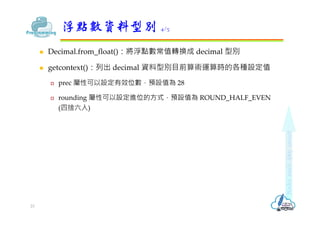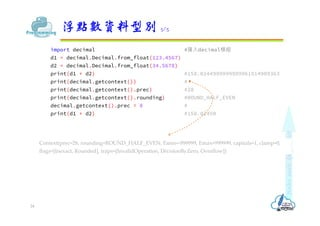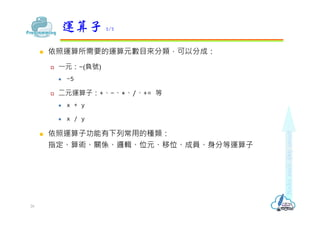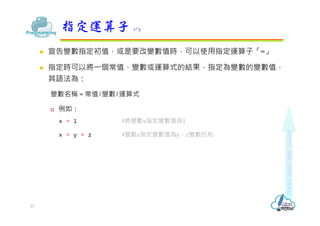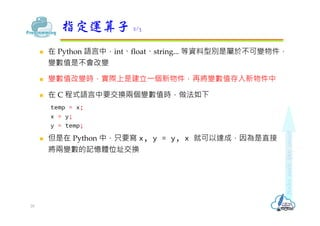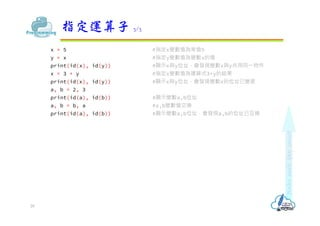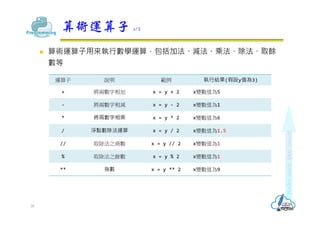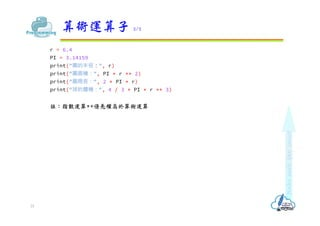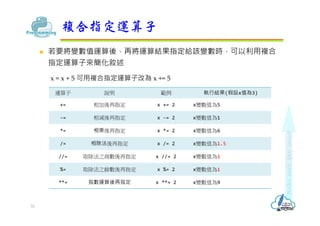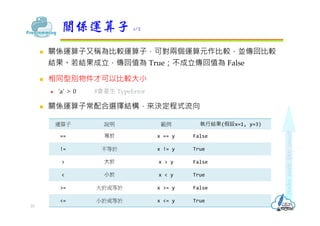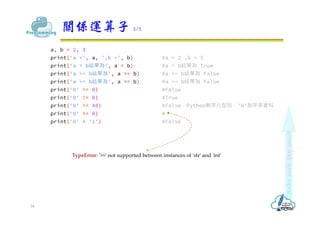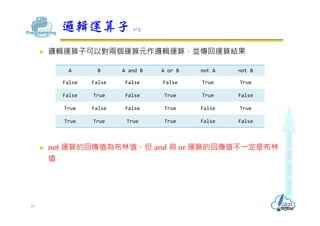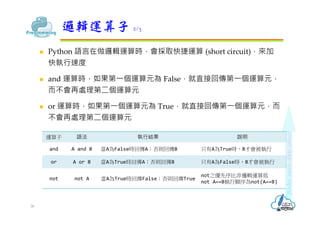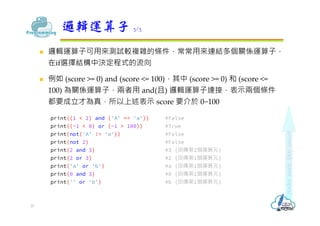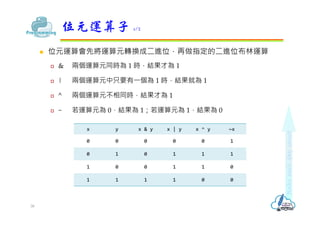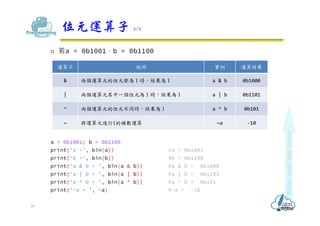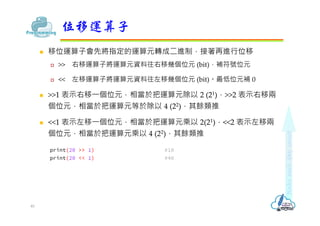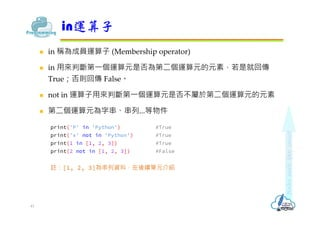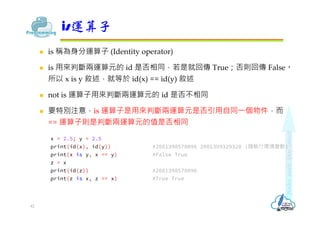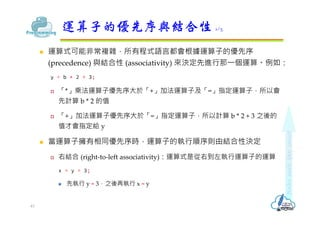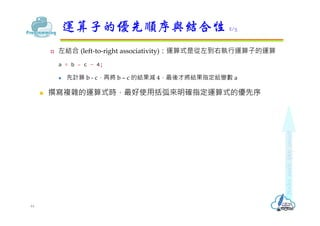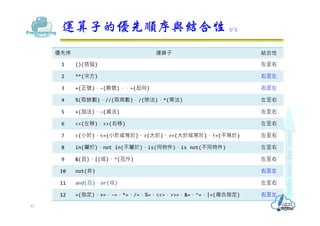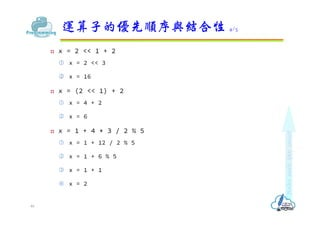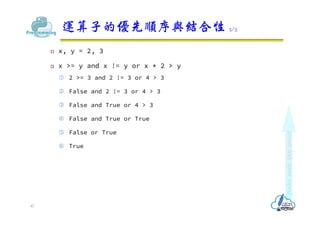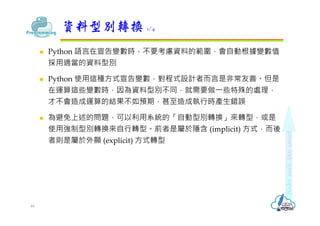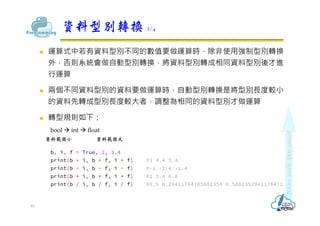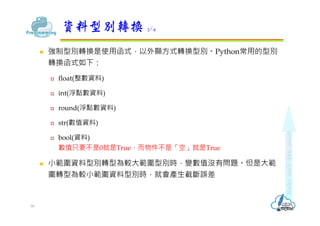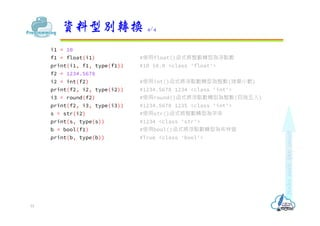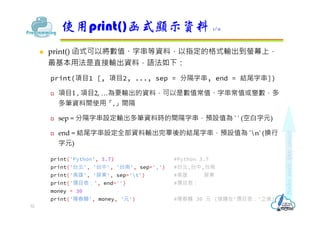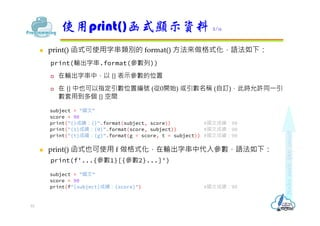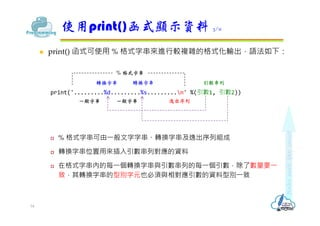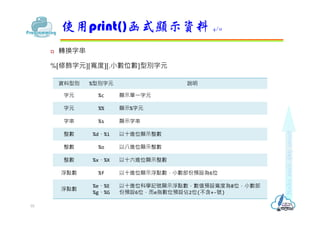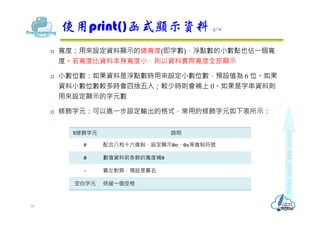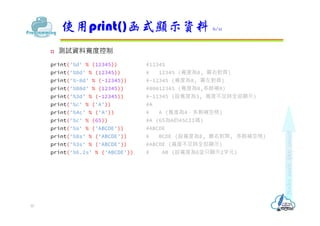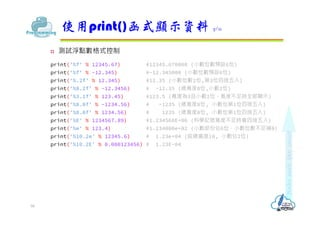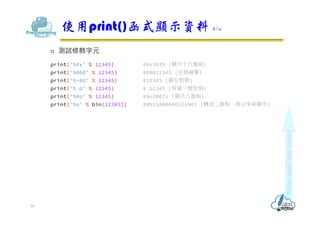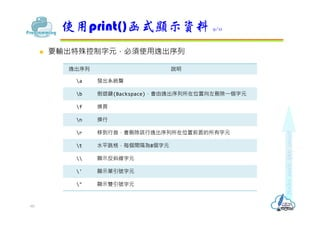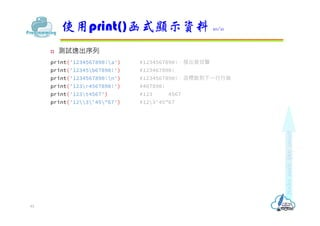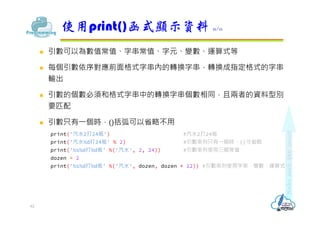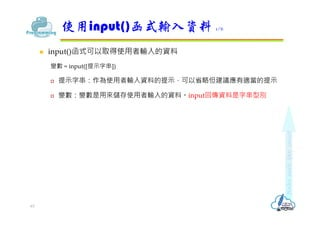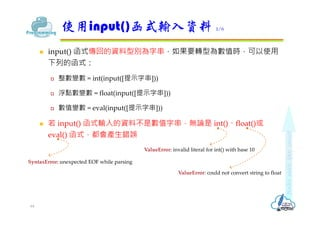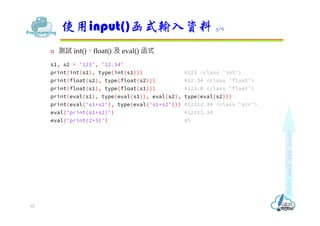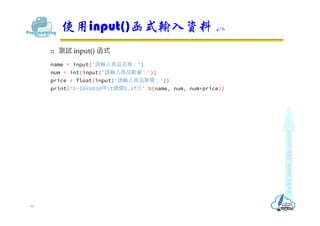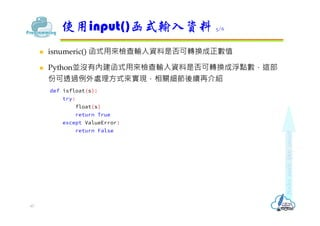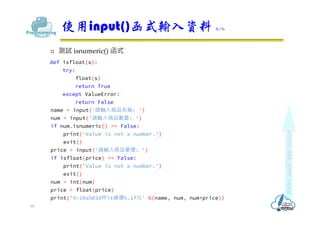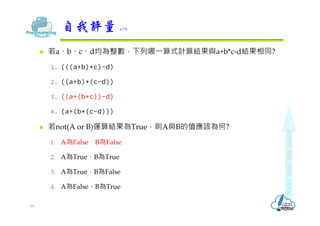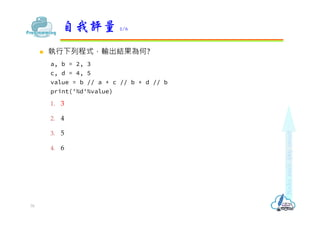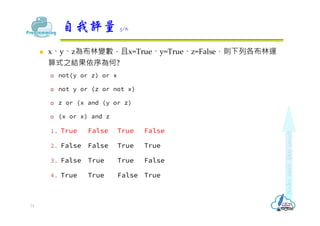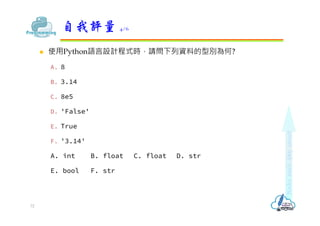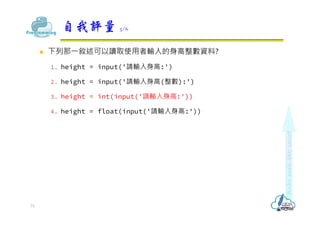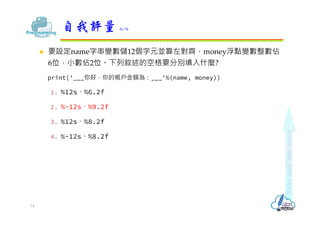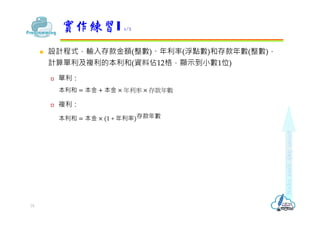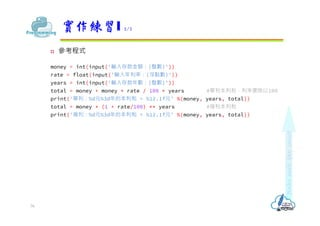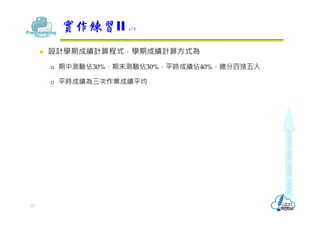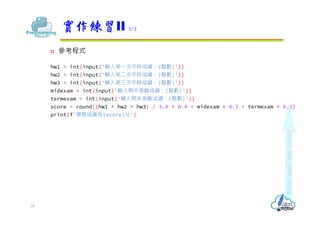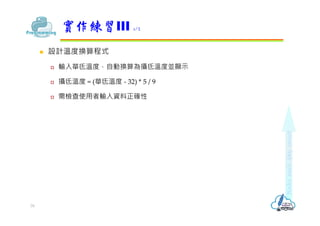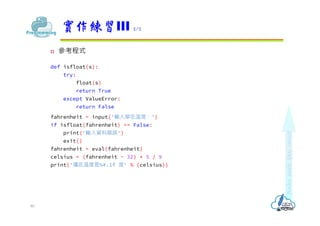1 sur 80
Publicité

### Python基本資料運算

1. Python基本資料運算 Revised on August 14, 2021  內建資料型別  常值  變數  運算子與運算式  資料型別轉換  使用print()函式  使用input()函式
2.  資料處理是應用程式的核⼼作業之⼀，不論該資料是使用者從鍵盤輸 入，還是來自檔案或網路等其它資料源  運算是指將原始資料經過特定處理，並產生輸出結果  設計資料處理應用程式時，必須考量資料驗證與轉換 資料處理 2
3.  程式經常需要處理不同類型的資料  課程名稱是字串資料  修課學生人數是整數資料  修課學生的平均成績是浮點數資料  程式使用記憶體資源儲存運算資料，設計程式時必須依據資料的類型 及可能的大小，選用合適資料型別，才能順利運作又不浪費系統資源  Python 的標準函式庫中，提供許多內建的資料型別，可以做為程式中 處理資料的型別 內建資料型別 1/4 3
4.  數值資料型別 (Numeric type) 提供 int、float 等可以處理數值的資料型別  文字序列資料型別 (Text sequence type) 提供 str 資料型別來處理字串文字資料  序列資料型別 (Sequence type) 提供 list、tuple 和 range 三種資料型別，可以處理整組系列的資料  映射資料型別 (Mapping type) 提供 dict 資料型別，可以使用關鍵字查詢相對應的資料  本單元先介紹數值及文字序列這二類資料型別 內建資料型別 2/4 4
5.  Python的內建資料型別，都是以物件的形式實作而成  在物件導向程式中，特性類似的物件會歸類成同⼀個類別 (Class)，而 物件 (Object) 是由類別所實作而成的實體 (Instance)  例如車牌 ABC-1234 和車牌 ABC-2345 的車子，雖然都是「車子」類別的 實體，但各為不同的物件  物件屬性 (attribute/property) 是指物件具備的性質，例如車子的排氣 量、顏色、廠牌  物件方法 (method) 是指物件具備的功能，例如汽車可以發動、加速、 剎車、換檔  「.」運算子用來取得物件的屬性值或執行方法。例如  myCar.color #車子顏色值  myCar.shift('D') #換D檔 內建資料型別 3/4 5
6.  type() 函式：取得物件的類型  isinstance() 函式：可讓您判斷所預期的某個值是否為特定資料類型， 如果預期正確，則會傳回值 True；如果不正確，則為 False print(type('7')) #<class 'str'> print(type(7)) #<class 'int'> print(type(7.1)) #<class 'float'> print(isinstance('7', str)) #True print(isinstance(7, float)) #False print(isinstance(7.1, float)) #True 內建資料型別 4/4 6
7.  「常值」(或稱字面值) 是指資料本身的值不需要經過宣告，寫在指令 敘述中電腦就可以直接處理的數值或字串資料  例如：3、'three'、True 等  Python 提供常用的常值有：  整數常值用來表示整數  布林常值只有 True (真) 和 False (假) 兩種值  浮點常值是用來表示帶有小數點數字的資料  字串常值是用來表示連續的字元 常值(Literal) 1/5 7
8.  整數常值  整數 (Integral) 常值是指沒有帶小數位數的整數數值，例如年齡  Python語言可以使用 10 進制、2 進制、8 進制及 16 進制來處理整數常值  ⼗進制：程式中⼀連串的數字，會預設為⼗進制的 int 整數資料，例如 123  二進制：由數字 0 和 1 所組成，必須以 0b 開頭，例如 0b01111011  八進制：由數字 0 ~ 7 所組成，必須以 0o 開頭，例如 0o173  ⼗六進制：由數字 0 ~ 9 和字⺟ a ~ f 所組成，必須以 0x 開頭，例如 0x7b print(123) #123 print(0b01111011) #123 print(0o173) #123 print(0x7b) #123 常值(Literal) 2/5 8
9.  布林常值  布林 (Boolean) 常值只有 True 和 False 兩種值，True 代表真、False 代表 假，常用於程式的邏輯判斷，例如成績及格/不及格  浮點數常值  浮點數 (Floating point) 常值又稱為實數常值，需要用到帶小數點的數值時， 例如圓周率，就必須使用浮點數常值  浮點數有兩種表示方式：  小數點表示法，例如 3.14159  科學記號，例如 2.34e+5，表示 2.34×105，即 234000.0 print(12.345) #12.345 print(1.2345e2) #123.45 print(1.2345e8) #123450000.0 print(1.2345e-2) #0.012345 常值(Literal) 3/5 9
10.  字串常值  字串常值是由⼀個或⼀個以上的連串字元，頭尾使用單引號「'」或雙引號 「"」括住  例如 'Welcome'、'True'、'1234’ 等，都是屬於字串常值  在 Python 中字串常值是屬於 str 類別 print('Python') #顯示字串常值'Python' print('3.7') #顯示字串常值'3.7' print("This's a book.") #字串常值中有單引號時用"..." print('"Hi!" says Jack.') #字串常值中有雙引號時用'...' print('0') #顯示字串常值'0' 註：Python沒有字元型別，'0'為str類別 常值(Literal) 5/5 10
11.  字串中的特殊字元必須使用逸出字元  使用 '''起始與結束的內容會保留原來格式 content = 'Hello Pythonn中文字測試nWelcome' content2 = '''Hello Python 中文字測試 Welcome''' 常值(Literal) 5/5 11 脫逸字元 說明 脫逸字元 說明 ' 單引號字元 " 雙引號 反斜線字元 n 換行 r 游標移至列首 t Tab鍵 v 垂直定位 a 響鈴 b 後退鍵 f 換頁 x 16進位字元碼 o 8進位字元碼
12.  在程式設計時所使用的變數、函式、類別等都會給予名稱，這些名稱 稱為「識別字 (Identifier)」  識別字在程式中必須是唯⼀的名稱，命名規則如下：  第⼀個字元限用英文字⺟或底線  後續可由大小寫英文字⺟、阿拉伯數字和底線(_)所組成  大小寫字⺟視為不相同的字元，所以 ok、Ok、OK 為三個不同的識別字  不能使用保留字 (Reserved word) 當作識別字  name   7Eleven   B&Q   and  識別字 12  and是Python的保留字
13.  保留字又稱關鍵字 (Keyword)，是 Python 語言具特定用途的識別字  Python3.x 定義的保留字： and as assert async await break class continue def del elif else except False finally for from global if import in is lambda None nonlocal not or pass raise True return try while with yield 保留字 13
14.  變數 (Variable) 是隨時可以改變內容的容器名稱，使用變數可以使程 式更靈活處理資料  程式中可以直接選擇未使用且不是Python保留字的名稱來建立變數， 並指定變數值。語法如下：  變數 = 變數值 a = 3  變數1, 變數2 [, 變數3…] = 變數值1, 變數值2 [,變數值3…] a, b = 3, 5  變數1 [= 變數2 = 變數3…] = 變數值 a = b = 10  Python 程式是採用動態型別，程式在直譯時才會根據變數值，宣告成 適當的資料型別 變數宣告 1/3 14
15.  Python 不需要轉換任何資料，可以隨時更動變數的資料型別，只要使用 指定運算子「=」，就能重新指定變數所參考的物件 x = 'a string' x = 7 x = False  變數的名稱除了要遵循識別字的命名規則外，要使用易懂而且有意義的 名稱，以提高程式的可讀性  Python3 以上版本支援中文識別字名稱，但是 Python 鼓勵開源共享，仍 然建議不要使用中文變數  若變數不再使用，可以使用 del 指令來刪除變數，如此可以將佔用的記 憶體釋放出來 變數宣告 2/3 15
16. power1 = power2 = 100 #宣告power1和power2變數，變數值都為100 total = 1.23456E+6 #宣告total變數，值為浮點數1234560.0 print(yesNo) #y print(passScore, maxScore, minScore)#60 100 0 print(power1, power2) #100 100 print(total) #1234560.0 del total print(total) #執行會產生錯誤，因為變數已經刪除 變數宣告 3/3 16 NameError: name 'total' is not defined
17.  Python 內建的整數型別是屬於 int 類別，⻑度不受限制，除非電腦的 記憶體不足  可以使用 Python 內建函式來處理整數資料  bin() 函式：將⼗進制整數轉換成二進制  oct() 函式：將⼗進制整數轉換成八進制  hex() 函式：將⼗進制整數轉換成⼗六進制  int() 函式：將其他進制的整數轉換成⼗進制，也可以將數字字串轉換成 int 整數 整數資料型別 1/2 17
18. print(type(12)) #<class 'int'> print(bin(12)) #0b1100 print(oct(12)) #0o14 print(hex(12)) #0xc print('12' * 4) #12121212 print(type('12')) #<class 'str'> print(int('12') * 4) #48 整數資料型別 2/2 18
19.  布林值是屬於 bool 類別，而 bool 類別是 int 類別的子類別  使用 bool() 函式可以其它物件資料轉成布林常值 True 和 False  使用 bool() 函式轉型時，數值只要不是 0 就是 True，而物件不是「空」 就是 True b = False #宣告b為布林變數，變數值為False print(type(b)) #顯示布林變數b的類別 <class 'bool'> print(type(1)) #顯示整數常值1的類別 <class 'int'> print(bool(1)) #使用bool函式將1轉成布林值 True print(bool(-1)) #使用bool函式將-1轉成布林值 True print(bool(0)) #使用bool函式將0轉成布林值 False print(bool('Python')) #使用bool函式將'Python'轉成布林值 True 布林資料型別 19
20.  float 浮點數資料型別，屬於 float 類別  float() 函式：將整數或數值字串轉換成浮點數  is_integer() 方法：檢查浮點數是否小數位為 0，傳回值為 True (是整數)和 False (非整數)  round() 函式：將浮點數的小數部分，指定位數做四捨五入 語法為：round (浮點數[，小數位數])，如果不指定小數位數時，就預設四 捨五入為整數 f, i = 1.2345, 12345 print(type(f)) #顯示浮點數變值f的類別 <class 'float'> f2 = float(i) #用float函式將整數變數i轉成浮點數 print(float(i)) #顯示f2的變數值 12345.0 print(float.is_integer(f)) #用is integer()方法檢查變數f是否為整數 False print(float.is_integer(f2)) #檢查變數f2是否為整數 True print(round(f, 2)) #用round()函式將變數f四捨五入到小數二位 1.23 print(round(f)) #用round()函式將變數f四捨五入到整數 1 浮點數資料型別 1/5 20
21.  decimal 資料型別同樣處理浮點值，但準確度較浮點數高  使用 decimal 資料型別前要使用 import 指令引用 decimal 模組  使用 Decimal() 方法，可宣告 decimal 型別資料  Decimal() 方法的引數可以是整數常值或字串、浮點數字串  decimal.Decimal (整數常值|整數字串|浮點數字串)  如果要指定小數的有效位數時，在宣告時採用浮點數字串的格式 浮點數資料型別 2/5 21
22. import decimal #匯入decimal模組 f1, f2 = 10.0, 3.0 #宣告f1、f2變數並指定變數值為浮點數10.0和3.0 d1 = decimal.Decimal(10) #宣告d1為decimal型別變數，值為整數常值10 d2 = decimal.Decimal('3.0') #宣告d2為decimal型別變數，值為字串常值'3.0' print(type(d1)) #<class 'decimal.Decimal'> print(f1 / f2) #3.3333333333333335 print(d1 / d2) #3.333333333333333333333333333 d3 = decimal.Decimal('2.345') #宣告d3為decimal型別變數，值為字串常值'2.345' d4 = decimal.Decimal('6.78') print(d3 + d4) #9.125 print(d3 * d4) #15.89910 浮點數資料型別 3/5 22
23.  Decimal.from_float()：將浮點數常值轉換成 decimal 型別  getcontext()：列出 decimal 資料型別目前算術運算時的各種設定值  prec 屬性可以設定有效位數，預設值為 28  rounding 屬性可以設定進位的方式，預設值為 ROUND_HALF_EVEN (四捨六入) 浮點數資料型別 4/5 23
24. import decimal #匯入decimal模組 d1 = decimal.Decimal.from_float(123.4567) d2 = decimal.Decimal.from_float(34.5678) print(d1 + d2) #158.0244999999999961914909363 print(decimal.getcontext()) # print(decimal.getcontext().prec) #28 print(decimal.getcontext().rounding) #ROUND_HALF_EVEN decimal.getcontext().prec = 8 # print(d1 + d2) #158.02450 浮點數資料型別 5/5 24 Context(prec=28, rounding=ROUND_HALF_EVEN, Emin=-999999, Emax=999999, capitals=1, clamp=0, flags=[Inexact, Rounded], traps=[InvalidOperation, DivisionByZero, Overflow])
25.  運算式是由運算元 (operand) 與運算子 (operator) 所組成的計算式  運算子是指對運算元做特定運算的符號，例如 +、-、*、/ 等  運算元是運算的對象，運算元可以為變數、常值或是運算式 num = 5 + 3 - x 運算子 1/2 運算元 運算元 運算元 運算元 運算子 運算子 運算子 25
26.  依照運算所需要的運算元數目來分類，可以分成：  ⼀元：-(負號)  -5  二元運算子：+、-、*、/、+= 等  x + y  x / y  依照運算子功能有下列常用的種類： 指定、算術、關係、邏輯、位元、移位、成員、身分等運算子 運算子 2/2 26
27.  宣告變數指定初值，或是要改變數值時，可以使用指定運算子「=」  指定時可以將⼀個常值、變數或運算式的結果，指定為變數的變數值， 其語法為： 變數名稱 = 常值|變數|運算式  例如： x = 1 #將變數x指定變數值為1 x = y + z #變數x指定變數值為y、z變數的和 指定運算子 1/3 27
28.  在 Python 語言中，int、float、string... 等資料型別是屬於不可變物件， 變數值是不會改變  變數值改變時，實際上是建立⼀個新物件，再將變數值存入新物件中  在 C 程式語言中要交換兩個變數值時，做法如下 temp = x; x = y; y = temp;  但是在 Python 中，只要寫 x, y = y, x 就可以達成，因為是直接 將兩變數的記憶體位址交換 指定運算子 2/3 28
29. x = 5 #指定x變數值為常值5 y = x #指定y變數值為變數x的值 print(id(x), id(y)) #顯示x與y位址，會發現變數x與y共用同⼀物件 x = 3 + y #指定x變數值為運算式3+y的結果 print(id(x), id(y)) #顯示x與y位址，會發現變數x的位址已變更 a, b = 2, 3 print(id(a), id(b)) #顯示變數a,b位址 a, b = b, a #a,b變數值交換 print(id(a), id(b)) #顯示變數a,b位址，會發現a,b的位址已互換 指定運算子 3/3 29
30.  算術運算子用來執行數學運算，包括加法、減法、乘法、除法、取餘 數等 算術運算子 1/2 運算子 說明 範例 執行結果(假設y值為3) + 將兩數字相加 x = y + 2 x變數值為5 - 將兩數字相減 x = y - 2 x變數值為1 * 將兩數字相乘 x = y * 2 x變數值為6 / 浮點數除法運算 x = y / 2 x變數值為1.5 // 取除法之商數 x = y // 2 x變數值為1 % 取除法之餘數 x = y % 2 x變數值為1 ** 指數 x = y ** 2 x變數值為9 30
31. r = 6.4 PI = 3.14159 print("圓的半徑：", r) print("圓面積：", PI * r ** 2) print("圓周⻑：", 2 * PI * r) print("球的體積：", 4 / 3 * PI * r ** 3) 註：指數運算**優先權高於算術運算 算術運算子 2/2 31
32.  若要將變數值運算後，再將運算結果指定給該變數時，可以利用複合 指定運算子來簡化敘述 x = x + 5 可用複合指定運算子改為 x += 5 複合指定運算子 運算子 說明 範例 執行結果(假設x值為3) += 相加後再指定 x += 2 x變數值為5 -= 相減後再指定 x -= 2 x變數值為1 *= 相乘後再指定 x *= 2 x變數值為6 /= 相除法後再指定 x /= 2 x變數值為1.5 //= 取除法之商數後再指定 x //= 2 x變數值為1 %= 取除法之餘數後再指定 x %= 2 x變數值為1 **= 指數運算後再指定 x **= 2 x變數值為9 32
33.  關係運算子又稱為比較運算子，可對兩個運算元作比較，並傳回比較 結果。若結果成立，傳回值為 True；不成立傳回值為 False  相同型別物件才可以比較大小  'a' > 0 #會產生 TypeError  關係運算子常配合選擇結構，來決定程式流向 關係運算子 1/2 運算子 說明 範例 執行結果(假設x=1, y=3) == 等於 x == y False != 不等於 x != y True > 大於 x > y False < 小於 x < y True >= 大於或等於 x >= y False <= 小於或等於 x <= y True 33
34. a, b = 2, 3 print('a =', a, ',b =', b) #a = 2 ,b = 3 print('a < b結果為', a < b) #a < b結果為 True print('a >= b結果為', a >= b) #a >= b結果為 False print('a == b結果為', a == b) #a == b結果為 False print('0' == 0) #False print('0' != 0) #True print('0' == 48) #False，Python無字元型別，'0'為字串資料 print('0' >= 0) # print('0' < '1') #False 關係運算子 2/2 34 TypeError: '>=' not supported between instances of 'str' and 'int'
35.  邏輯運算子可以對兩個運算元作邏輯運算，並傳回運算結果  not 運算的回傳值為布林值，但 and 與 or 運算的回傳值不⼀定是布林 值 邏輯運算子 1/3 A B A and B A or B not A not B False False False False True True False True False True True False True False False True False True True True True True False False 35
36.  Python 語言在做邏輯運算時，會採取快捷運算 (short circuit)，來加 快執行速度  and 運算時，如果第⼀個運算元為 False，就直接回傳第⼀個運算元， 而不會再處理第二個運算元  or 運算時，如果第⼀個運算元為 True，就直接回傳第⼀個運算元，而 不會再處理第二個運算元 邏輯運算子 2/3 運算子 語法 執行結果 說明 and A and B 當A為False時回傳A；否則回傳B 只有A為True時，B才會被執行 or A or B 當A為True時回傳A；否則回傳B 只有A為False時，B才會被執行 not not A 當A為True時回傳False；否則回傳True not之優先序比非邏輯運算低 not A==B執行順序為not(A==B) 36
37.  邏輯運算子可用來測試較複雜的條件，常常用來連結多個關係運算子， 在if選擇結構中決定程式的流向  例如 (score >= 0) and (score <= 100)，其中 (score >= 0) 和 (score <= 100) 為關係運算子，兩者用 and(且) 邏輯運算子連接，表示兩個條件 都要成立才為真，所以上述表示 score 要介於 0~100 print((1 < 2) and ('A' == 'a')) #False print((-1 < 0) or (-1 > 100)) #True print(not('A' != 'a')) #False print(not 2) #False print(2 and 3) #3 (回傳第2個運算元) print(2 or 3) #2 (回傳第1個運算元) print('a' or 'b') #a (回傳第1個運算元) print(0 and 3) #0 (回傳第1個運算元) print('' or 'b') #b (回傳第1個運算元) 邏輯運算子 3/3 37
38.  位元運算會先將運算元轉換成二進位，再做指定的二進位布林運算  & 兩個運算元同時為 1 時，結果才為 1  | 兩個運算元中只要有⼀個為 1 時，結果就為 1  ^ 兩個運算元不相同時，結果才為 1  ~ 若運算元為 0，結果為 1；若運算元為 1，結果為 0 位元運算子 1/2 x y x & y x | y x ^ y ~x 0 0 0 0 0 1 0 1 0 1 1 1 1 0 0 1 1 0 1 1 1 1 0 0 38
39.  若a = 0b1001，b = 0b1100 a = 0b1001; b = 0b1100 print('a =', bin(a)) #a = 0b1001 print('b =', bin(b)) #b = 0b1100 print('a & b = ', bin(a & b)) #a & b = 0b1000 print('a | b = ', bin(a | b)) #a | b = 0b1101 print('a ^ b = ', bin(a ^ b)) #a ^ b = 0b101 print('~a = ', ~a) #~a = -10 運算子 說明 實例 運算結果 & 兩個運算元的位元都為 1 時，結果為 1 a & b 0b1000 | 兩個運算元其中一個位元為 1 時，結果為 1 a | b 0b1101 ^ 兩個運算元的位元不同時，結果為 1 a ^ b 0b101 ~ 將運算元進行1的補數運算 ~a -10 位元運算子 2/2 39
40.  移位運算子會先將指定的運算元轉成二進制，接著再進行位移  >> 右移運算子將運算元資料往右移幾個位元 (bit)，補符號位元  << 左移運算子將運算元資料往左移幾個位元 (bit)。最低位元補 0  >>1 表示右移⼀個位元，相當於把運算元除以 2 (21)，>>2 表示右移兩 個位元，相當於把運算元等於除以 4 (22)，其餘類推  <<1 表示左移⼀個位元，相當於把運算元乘以 2(21)，<<2 表示左移兩 個位元，相當於把運算元乘以 4 (22)，其餘類推 print(20 >> 1) #10 print(20 << 1) #40 位移運算子 40
41.  in 稱為成員運算子 (Membership operator)  in 用來判斷第⼀個運算元是否為第二個運算元的元素，若是就回傳 True；否則回傳 False。  not in 運算子用來判斷第⼀個運算元是否不屬於第二個運算元的元素  第二個運算元為字串、串列...等物件 print('P' in 'Python') #True print('x' not in 'Python') #True print(1 in [1, 2, 3]) #True print(2 not in [1, 2, 3]) #False 註：[1, 2, 3]為串列資料，在後續單元介紹 in運算子 41
42.  is 稱為身分運算子 (Identity operator)  is 用來判斷兩運算元的 id 是否相同，若是就回傳 True；否則回傳 False。 所以 x is y 敘述，就等於 id(x) == id(y) 敘述  not is 運算子用來判斷兩運算元的 id 是否不相同  要特別注意，is 運算子是用來判斷兩運算元是否引用自同⼀個物件，而 == 運算子則是判斷兩運算元的值是否相同 x = 2.5; y = 2.5 print(id(x), id(y)) #2081398578096 2081399329328 (隨執行環境變動) print(x is y, x == y) #False True z = x print(id(z)) #2081398578096 print(z is x, z == x) #True True is運算子 42
43.  運算式可能非常複雜，所有程式語言都會根據運算子的優先序 (precedence) 與結合性 (associativity) 來決定先進行那⼀個運算。例如： y = b * 2 + 3;  「*」乘法運算子優先序大於「+」加法運算子及「=」指定運算子，所以會 先計算 b * 2 的值  「+」加法運算子優先序大於「=」指定運算子，所以計算 b * 2 + 3 之後的 值才會指定給 y  當運算子擁有相同優先序時，運算子的執行順序則由結合性決定  右結合 (right-to-left associativity)：運算式是從右到左執行運算子的運算 x = y = 3;  先執行 y = 3，之後再執行 x = y 運算子的優先序與結合性 1/5 43
44.  左結合 (left-to-right associativity)：運算式是從左到右執行運算子的運算 a = b – c - 4;  先計算 b - c，再將 b – c 的結果減 4，最後才將結果指定給變數 a  撰寫複雜的運算式時，最好使用括弧來明確指定運算式的優先序 運算子的優先順序與結合性 2/5 44
45. 運算子的優先順序與結合性 3/5 45 優先序 運算子 結合性 1 ()(括弧) 左至右 2 **(次方) 右至左 3 +(正號)，-(負號)，，~(反向) 右至左 4 %(取餘數)，//(取商數)，/(除法)，*(乘法) 左至右 5 +(加法)，-(減法) 左至右 6 <<(左移)，>>(右移) 左至右 7 <(小於)，<=(小於或等於)，>(大於)，>=(大於或等於)，!=(不等於) 左至右 8 in(屬於)，not in(不屬於)，is(同物件)，is not(不同物件) 左至右 9 &(且)、|(或)、^(互斥) 左至右 10 not(非) 右至左 11 and(且)，or(或) 左至右 12 =(指定)，+=，-=，*=，/=，%=，<<=，>>=，&=，^=，|=(複合指定) 右至左
46.  x = 2 << 1 + 2  x = 2 << 3  x = 16  x = (2 << 1) + 2  x = 4 + 2  x = 6  x = 1 + 4 * 3 / 2 % 5  x = 1 + 12 / 2 % 5  x = 1 + 6 % 5  x = 1 + 1  x = 2 運算子的優先順序與結合性 4/5 46
47.  x, y = 2, 3  x >= y and x != y or x * 2 > y  2 >= 3 and 2 != 3 or 4 > 3  False and 2 != 3 or 4 > 3  False and True or 4 > 3  False and True or True  False or True  True 運算子的優先順序與結合性 5/5 47
48.  Python 語言在宣告變數時，不要考慮資料的範圍，會自動根據變數值 採用適當的資料型別  Python 使用這種方式宣告變數，對程式設計者而言是非常友善。但是 在運算這些變數時，因為資料型別不同，就需要做⼀些特殊的處理， 才不會造成運算的結果不如預期，甚至造成執行時產生錯誤  為避免上述的問題，可以利用系統的「自動型別轉換」來轉型，或是 使用強制型別轉換來自行轉型。前者是屬於隱含 (implicit) 方式，而後 者則是屬於外顯 (explicit) 方式轉型 資料型別轉換 1/4 48
49.  運算式中若有資料型別不同的數值要做運算時，除非使用強制型別轉換 外，否則系統會做自動型別轉換，將資料型別轉成相同資料型別後才進 行運算  兩個不同資料型別的資料要做運算時，自動型別轉換是將型別⻑度較小 的資料先轉成型別⻑度較大者，調整為相同的資料型別才做運算  轉型規則如下： bool  int  float b, i, f = True, 2, 3.4 print(b + i, b + f, i + f) #3 4.4 5.4 print(b - i, b - f, i - f) #-1 -2.4 -1.4 print(b * i, b * f, i * f) #2 3.4 6.8 print(b / i, b / f, i / f) #0.5 0.29411764705882354 0.5882352941176471 資料型別轉換 2/4 資料範圍小 資料範圍大 49
50.  強制型別轉換是使用函式，以外顯方式轉換型別。Python常用的型別 轉換函式如下：  float(整數資料)  int(浮點數資料)  round(浮點數資料)  str(數值資料)  bool(資料) 數值只要不是0就是True，而物件不是「空」就是True  小範圍資料型別轉型為較大範圍型別時，變數值沒有問題。但是大範 圍轉型為較小範圍資料型別時，就會產生截斷誤差 資料型別轉換 3/4 50
51. i1 = 10 f1 = float(i1) #使用float()函式將整數轉型為浮點數 print(i1, f1, type(f1)) #10 10.0 <class 'float'> f2 = 1234.5678 i2 = int(f2) #使用int()函式將浮點數轉型為整數(捨棄小數) print(f2, i2, type(i2)) #1234.5678 1234 <class 'int'> i3 = round(f2) #使用round()函式將浮點數轉型為整數(四捨五入) print(f2, i3, type(i3)) #1234.5678 1235 <class 'int'> s = str(i2) #使用str()函式將整數轉型為字串 print(s, type(s)) #1234 <class 'str'> b = bool(f1) #使用bool()函式將浮點數轉型為布林值 print(b, type(b)) #True <class 'bool'> 資料型別轉換 4/4 51
52.  print() 函式可以將數值、字串等資料，以指定的格式輸出到螢幕上， 最基本用法是直接輸出資料，語法如下： print(項目1 [, 項目2, ..., sep = 分隔字串, end = 結尾字串])  項目1 , 項目2, …為要輸出的資料，可以是數值常值、字串常值或變數，多 多筆資料間使用「,」間隔  sep = 分隔字串設定輸出多筆資料時的間隔字串，預設值為 ' ' (空白字元)  end = 結尾字串設定全部資料輸出完畢後的結尾字串，預設值為 'n' (換行 字元) print('Python', 3.7) #Python 3.7 print('台北', '台中', '台南', sep=',') #台北,台中,台南 print('高雄', '屏東', sep='t') #高雄 屏東 print('價目表：', end='') #價目表： money = 30 print('陽春麵', money, '元') #陽春麵 30 元 (接續在'價目表：'之後) 使用print()函式顯示資料 1/11 52
53.  print() 函式可使用字串類別的 format() 方法來做格式化，語法如下： print(輸出字串.format(參數列))  在輸出字串中，以 {} 表示參數的位置  在 {} 中也可以指定引數位置編號 (從0開始) 或引數名稱 (自訂)，此時允許同⼀引 數套用到多個 {} 空間 subject = "國文" score = 90 print("{}成績：{}".format(subject, score)) #國文成績：90 print("{1}成績：{0}".format(score, subject)) #國文成績：90 print("{t}成績：{g}".format(g = score, t = subject)) #國文成績：90  print() 函式也可使用 f 做格式化，在輸出字串中代入參數，語法如下： print(f'...{參數1}[{參數2}...]') subject = "國文" score = 90 print(f"{subject}成績：{score}") #國文成績：90 使用print()函式顯示資料 2/11 53
54.  print() 函式可使用 % 格式字串來進行較複雜的格式化輸出，語法如下： print('.........%d.........%s.........n' %(引數1, 引數2))  % 格式字串可由⼀般文字字串、轉換字串及逸出序列組成  轉換字串位置用來插入引數串列對應的資料  在格式字串內的每⼀個轉換字串與引數串列的每⼀個引數，除了數量要⼀ 致，其轉換字串的型別字元也必須與相對應引數的資料型別⼀致 使用print()函式顯示資料 3/11 一般字串 轉換字串 轉換字串 逸出序列 一般字串 引數串列 54 % 格式字串
55.  轉換字串 %[修飾字元][寬度][.小數位數]型別字元 使用print()函式顯示資料 4/11 資料型別 %型別字元 說明 字元 %c 顯示單⼀字元 字元 %% 顯示%字元 字串 %s 顯示字串 整數 %d、%i 以⼗進位顯示整數 整數 %o 以八進位顯示整數 整數 %x、%X 以⼗六進位顯示整數 浮點數 %f 以⼗進位顯示浮點數，小數部份預設為6位 浮點數 %e、%E %g、%G 以⼗進位科學記號顯示浮點數，數值預設寬度為8位，小數部 份預設6位，而e指數位預設佔2位(不含+-號) 55
56.  寬度：用來設定資料顯示的總寬度(即字數)，浮點數的小數點也佔⼀個寬 度。若寬度比資料本身寬度小，則以資料實際寬度全部顯示  小數位數：如果資料是浮點數時用來設定小數位數，預設值為 6 位。如果 資料小數位數較多時會四捨五入；較少時則會補上 0。如果是字串資料則 用來設定顯示的字元數  修飾字元：可以進⼀步設定輸出的格式，常用的修飾字元如下表所示： 使用print()函式顯示資料 5/11 %修飾字元 說明 # 配合八和⼗六進制，設定顯示0o、0x等進制符號 0 數值資料前多餘的寬度補0 - 靠左對齊，預設是靠右 空白字元 保留⼀個空格 56
57.  測試資料寬度控制 print('%d' % (12345)) #12345 print('%8d' % (12345)) # 12345 (寬度為8, 靠右對齊) print('%-8d' % (-12345)) #-12345 (寬度為8, 靠左對齊) print('%08d' % (12345)) #00012345 (寬度為8,多餘補0) print('%3d' % (-12345)) #-12345 (設寬度為3, 寬度不足時全部顯示) print('%c' % ('A')) #A print('%4c' % ('A')) # A (寬度為4，多剩補空格) print('%c' % (65)) #A (65為A的ASCII碼) print('%s' % ('ABCDE')) #ABCDE print('%8s' % ('ABCDE')) # BCDE (設寬度為8, 靠右對齊, 多餘補空格) print('%3s' % ('ABCDE')) #ABCDE (寬度不足時全部顯示) print('%6.2s' % ('ABCDE')) # AB (設寬度為6並只顯示2字元) 使用print()函式顯示資料 6/11 57
58.  測試浮點數格式控制 print('%f' % 12345.67) #12345.670000 (小數位數預設6位) print('%f' % -12.345) #-12.345000 (小數位數預設6位) print('%.2f' % 12.345) #12.35 (小數位數2位,第3位四捨五入) print('%8.2f' % -12.3456) # -12.35 (總寬度8位,小數2位) print('%3.1f' % 123.45) #123.5 (寬度為3且小數1位，寬度不足時全部顯示) print('%8.0f' % -1234.56) # -1235 (總寬度8位, 小數位第1位四捨五入) print('%8.0f' % 1234.56) # 1235 (總寬度8位, 小數位第1位四捨五入) print('%E' % 1234567.89) #1.234568E+06 (科學記號寬度不足時會四捨五入) print('%e' % 123.4) #1.234000e+02 (小數部份佔6位，小數位數不足補0) print('%10.2e' % 12345.6) # 1.23e+04 (設總寬度10, 小數佔2位) print('%10.2E' % 0.000123456) # 1.23E-04 使用print()函式顯示資料 7/11 58
59.  測試修飾字元 print('%#x' % 12345) #0x3039 (顯示⼗六進制) print('%08d' % 12345) #00012345 (空格補零) print('%-8d' % 12345) #12345 (靠左對齊) print('% d' % 12345) # 12345 (保留⼀個空格) print('%#o' % 12345) #0o30071 (顯示八進制) print('%s' % bin(12345)) #0b11000000111001 (轉成二進制，再以字串顯示) 使用print()函式顯示資料 8/11 59
60.  要輸出特殊控制字元，必須使用逸出序列 使用print()函式顯示資料 9/11 逸出序列 說明 a 發出系統聲 b 倒退鍵(Backspace)，會由逸出序列所在位置向左刪除⼀個字元 f 換頁 n 換行 r 移到行首，會刪除該行逸出序列所在位置前面的所有字元 t 水平跳格，每個間隔為8個字元 顯示反斜線字元 ' 顯示單引號字元 " 顯示雙引號字元 60
61.  測試逸出序列 print('1234567890!a') #1234567890!，發出音效聲 print('12345b67890!') #123467890! print('1234567890!n') #1234567890!，游標跳到下⼀行行首 print('123r4567890!') #467890! print('123t4567') #123 4567 print('123'45"67') #123'45"67 使用print()函式顯示資料 10/11 61
62.  引數可以為數值常值、字串常值、字元、變數、運算式等  每個引數依序對應前面格式字串內的轉換字串，轉換成指定格式的字串 輸出  引數的個數必須和格式字串中的轉換字串個數相同，且兩者的資料型別 要匹配  引數只有⼀個時，()括弧可以省略不用 print('汽水2打24瓶') #汽水2打24瓶 print('汽水%d打24瓶' % 2) #引數串列只有⼀個時，()可省略 print('%s%d打%d瓶' %('汽水', 2, 24)) #引數串列使用三個常值 dozen = 2 print('%s%d打%d瓶' %('汽水', dozen, dozen * 12)) #引數串列使用字串、變數、運算式 使用print()函式顯示資料 11/11 62
63.  input()函式可以取得使用者輸入的資料 變數 = input([提示字串])  提示字串：作為使用者輸入資料的提示，可以省略但建議應有適當的提示  變數：變數是用來儲存使用者輸入的資料。input回傳資料是字串型別 使用input()函式輸入資料 1/6 63
64.  input() 函式傳回的資料型別為字串，如果要轉型為數值時，可以使用 下列的函式：  整數變數 = int(input([提示字串]))  浮點數變數 = float(input([提示字串]))  數值變數 = eval(input([提示字串]))  若 input() 函式輸入的資料不是數值字串，無論是 int()、float()或 eval() 函式，都會產生錯誤 使用input()函式輸入資料 2/6 64 ValueError: invalid literal for int() with base 10 ValueError: could not convert string to float SyntaxError: unexpected EOF while parsing
65.  測試 int()、float() 及 eval() 函式 s1, s2 = '123', '12.34' print(int(s1), type(int(s1))) #123 <class 'int'> print(float(s2), type(float(s2))) #12.34 <class 'float'> print(float(s1), type(float(s1))) #123.0 <class 'float'> print(eval(s1), type(eval(s1)), eval(s2), type(eval(s2))) print(eval('s1+s2'), type(eval('s1+s2'))) #12312.34 <class 'str'> eval('print(s1+s2)') #12312.34 eval('print(2+3)') #5 使用input()函式輸入資料 3/6 65
66.  測試 input() 函式 name = input('請輸入商品名稱：') num = int(input('請輸入商品數量：')) price = float(input('請輸入商品單價：')) print('%-10s%03d件t總價%.1f元' %(name, num, num*price)) 使用input()函式輸入資料 4/6 66
67.  isnumeric() 函式用來檢查輸入資料是否可轉換成正數值  Python並沒有內建函式用來檢查輸入資料是否可轉換成浮點數，這部 份可透過例外處理方式來實現，相關細節後續再介紹 def isfloat(s): try: float(s) return True except ValueError: return False 使用input()函式輸入資料 5/6 67
68.  測試 isnumeric() 函式 def isfloat(s): try: float(s) return True except ValueError: return False name = input('請輸入商品名稱: ') num = input('請輸入商品數量: ') if num.isnumeric() == False: print('Value is not a number.') exit() price = input('請輸入商品單價: ') if isfloat(price) == False: print('Value is not a number.') exit() num = int(num) price = float(price) print('%-10s%03d件t總價%.1f元' %(name, num, num*price)) 使用input()函式輸入資料 6/6 68
69.  若a、b、c、d均為整數，下列哪⼀算式計算結果與a+b*c-d結果相同? 1. (((a+b)*c)-d) 2. ((a+b)*(c-d)) 3. ((a+(b*c))-d) 4. (a+(b*(c-d)))  若not(A or B)運算結果為True，則A與B的值應該為何? 1. A為False，B為False 2. A為True，B為True 3. A為True，B為False 4. A為False，B為True 自我評量 1/6 69
70.  執行下列程式，輸出結果為何? a, b = 2, 3 c, d = 4, 5 value = b // a + c // b + d // b print('%d'%value) 1. 3 2. 4 3. 5 4. 6 自我評量 2/6 70
71.  x、y、z為布林變數，且x=True、y=True、z=False，則下列各布林運 算式之結果依序為何?  not(y or z) or x  not y or (z or not x)  z or (x and (y or z)  (x or x) and z 1. True False True False 2. False False True True 3. False True True False 4. True True False True 自我評量 3/6 71
72.  使用Python語言設計程式時，請問下列資料的型別為何? A. 8 B. 3.14 C. 8e5 D. 'False' E. True F. '3.14' A. int B. float C. float D. str E. bool F. str 自我評量 4/6 72
73. 自我評量 5/6  下列那⼀敘述可以讀取使用者輸入的身高整數資料? 1. height = input('請輸入身高:') 2. height = input('請輸入身高(整數):') 3. height = int(input('請輸入身高:')) 4. height = float(input('請輸入身高:')) 73
74. 自我評量 6/6  要設定name字串變數儲12個字元並靠左對齊，money浮點變數整數佔 6位，小數佔2位。下列敘述的空格要分別填入什麼? print('___你好，你的帳戶金額為：___'%(name, money)) 1. %12s、%6.2f 2. %-12s、%9.2f 3. %12s、%8.2f 4. %-12s、%8.2f 74
75.  設計程式，輸入存款金額(整數)、年利率(浮點數)和存款年數(整數)， 計算單利及複利的本利和(資料佔12格，顯示到小數1位)  單利： 本利和 ൌ 本金 ൅ 本金 ൈ 年利率 ൈ 存款年數  複利： 本利和 ൌ 本金 ൈ ሺ1 + 年利率ሻ存款年數 實作練習I 1/2 75
76.  參考程式 money = int(input('輸入存款金額：(整數)')) rate = float(input('輸入年利率：(浮點數)')) years = int(input('輸入存款年數：(整數)')) total = money + money * rate / 100 * years #單利本利和，利率要除以100 print('單利：%d元%3d年的本利和 = %12.1f元' %(money, years, total)) total = money * (1 + rate/100) ** years #複利本利和 print('複利：%d元%3d年的本利和 = %12.1f元' %(money, years, total)) 實作練習I 2/2 76
77.  設計學期成績計算程式，學期成績計算方式為  期中測驗佔30%，期末測驗佔30%，平時成績佔40%，總分四捨五入  平時成績為三次作業成績平均 實作練習II 1/2 77
78.  參考程式 hw1 = int(input('輸入第⼀次平時成績：(整數)')) hw2 = int(input('輸入第二次平時成績：(整數)')) hw3 = int(input('輸入第三次平時成績：(整數)')) midexam = int(input('輸入期中測驗成績：(整數)')) termexam = int(input('輸入期末測驗成績：(整數)')) score = round((hw1 + hw2 + hw3) / 3.0 * 0.4 + midexam * 0.3 + termexam * 0.3) print(f'學期成績為{score}分') 實作練習II 2/2 78
79.  設計溫度換算程式  輸入華氐溫度，自動換算為攝氐溫度並顯示  攝氐溫度 = (華氐溫度 - 32) * 5 / 9  需檢查使用者輸入資料正確性 實作練習III 1/2 79
80.  參考程式 def isfloat(s): try: float(s) return True except ValueError: return False fahrenheit = input('輸入華氐溫度：') if isfloat(fahrenheit) == False: print('輸入資料錯誤') exit() fahrenheit = eval(fahrenheit) celsius = (fahrenheit - 32) * 5 / 9 print('攝氐溫度是%4.1f 度' % (celsius)) 實作練習III 2/2 80
Publicité